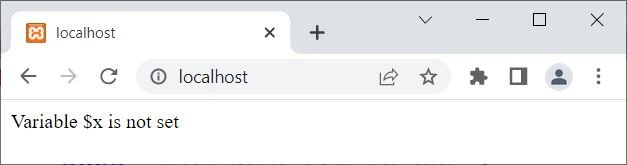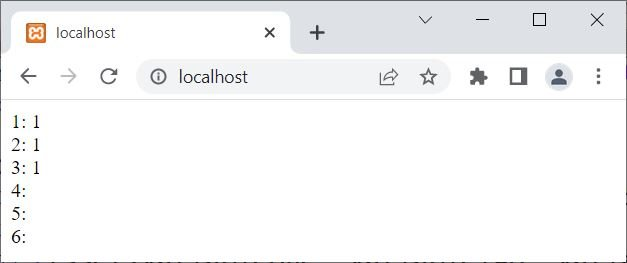# PHP isset(): Check if a variable is set

The PHP isset() function is used when we need to check whether a variable is set or not. For example:

```<?php
if(isset(\$x))
{
echo "Variable \\$x is set";
}
else
{
echo "Variable \\$x is not set";
}
?>```

Since the variable \$x is not set, the output of the above example is:## PHP isset() Syntax

The syntax of the isset() function in PHP is:

`isset(variableOne, variableTwo, variableThree, ..., variableN);`

At least one variable is required. Returns 1 if all given variables exist and are not NULL. Otherwise, it returns false or nothing. For example:

```<?php
\$x = 10;
\$y = 20;
\$z = 30;

echo "1: ", isset(\$x), "<BR>";
echo "2: ", isset(\$x, \$y), "<BR>";
echo "3: ", isset(\$x, \$y, \$z), "<BR>";
echo "4: ", isset(\$a), "<BR>";
echo "5: ", isset(\$x, \$a), "<BR>";
echo "6: ", isset(\$x, \$y, \$s), "<BR>";
?>```

The output of this PHP example using the isset() function is shown in the snapshot given below:In the above example, the returned value 1 by the isset() function can also be considered true.

## PHP isset() example

Consider the following PHP code as an example demonstrating the "isset()" function.

```<?php
\$a = 10;
\$b = 0;
\$c = "codescracker.com";
\$d = "PHP is Fun!";
\$e = 12.42;
\$f = true;
\$g = null;

if(isset(\$a))
echo "<p>The variable \\$a is set, with \$a</p>";
if(isset(\$b))
echo "<p>The variable \\$b is set, with \$b</p>";
if(isset(\$c))
echo "<p>The variable \\$c is set, with \$c</p>";
if(isset(\$d))
echo "<p>The variable \\$d is set, with \$d</p>";
if(isset(\$e))
echo "<p>The variable \\$e is set, with \$e</p>";
if(isset(\$f))
echo "<p>The variable \\$f is set, with \$f</p>";
if(isset(\$g))
echo "<p>The variable \\$g is set, with \$g</p>";
if(isset(\$z))
echo "<p>The variable \\$z is set, with \$z</p>";
if(isset(\$myvar))
echo "<p>The variable \\$myvar is set, with \$myvar</p>";
?>```

The output should be:

```The variable \$a is set, with 10

The variable \$b is set, with 0

The variable \$c is set, with codescracker.com

The variable \$d is set, with PHP is Fun!

The variable \$e is set, with 12.42

The variable \$f is set, with 1```

You can also print the counterpart, that is, the message when a variable is not set or defined, in this way:

```<?php
if(isset(\$myvar))
echo "<p>The variable \\$myvar is set, with \$myvar</p>";
else
echo "<p>The variable \\$myvar is not set</p>";
?>```

Or directly using:

```<?php
if(!isset(\$myvar))
echo "<p>The variable \\$myvar is not set</p>";
?>```

Since, in both the examples above, the variable \$myvar is not set, the output of these two codes should be:

`The variable \$myvar is not set`

PHP Online Test

« Previous Tutorial Next Tutorial »